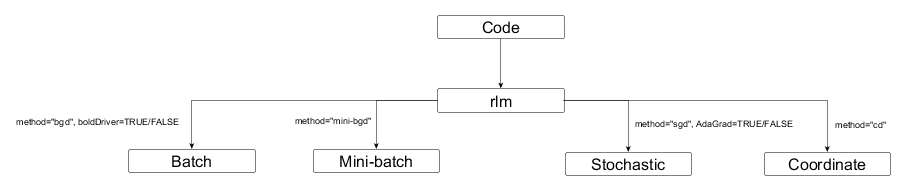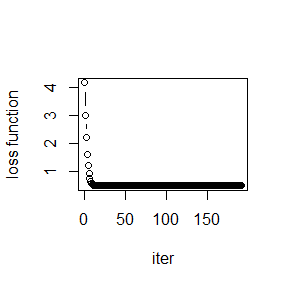# Introduction to RCANE

#### 2018-06-01

The goal of RCANE is to create an easy-to-use interface for gradient descent algorithms.

## Introduction

Rcane is a package which contains different numeric optimization algorithms for parameter estimation in linear regression.

4 algorithms are:

• Batch Gradient Descent

• Stochastic Gradient Descent

• Mini-batch Gradient Descent

• Coordinate Descent

Gradient descent is a first-order iterative optimization algorithm for finding minimum of a function. More information on Gradient Descent can be found here

## Package flow

The flow can be given as followsPackage flow

library(rcane)

## rlm for Batch Gradient Descent

### without boldDriver

rlm(formula = Petal.Length ~ Petal.Width, data = iris, method = "bgd")
#>
#> Call:
#> rlm(formula = Petal.Length ~ Petal.Width, data = iris, method = "bgd")
#>
#> Coefficients:
#> (Intercept) Petal.Width
#>    1.088651    2.226566

### with boldDriver

rlm(formula = Petal.Length ~ Petal.Width, data = iris, method = "bgd", boldDriver = TRUE)
#>
#> Call:
#> rlm(formula = Petal.Length ~ Petal.Width, data = iris, method = "bgd",
#>     boldDriver = TRUE)
#>
#> Coefficients:
#> (Intercept) Petal.Width
#>    1.083685    2.229799

## rlm for Stochastic Gradient Descent

### without AdaGrad

rlm(formula = Petal.Length ~ Petal.Width, data = iris, method = "sgd")
#>
#> Call:
#> rlm(formula = Petal.Length ~ Petal.Width, data = iris, method = "sgd")
#>
#> Coefficients:
#> (Intercept) Petal.Width
#>    1.095225    2.217139

### with Adagrad

rlm(formula = Petal.Length ~ Petal.Width, data = iris, method = "sgd", AdaGrad = TRUE)
#>
#> Call:
#> rlm(formula = Petal.Length ~ Petal.Width, data = iris, method = "sgd",
#>     AdaGrad = TRUE)
#>
#> Coefficients:
#> (Intercept) Petal.Width
#>   0.4488761   0.3931077

## rlm for Mini-batch Gradient Descent

rlm(formula = Petal.Length ~ Petal.Width, data = iris, method = "mini-bgd")
#>
#> Call:
#> rlm(formula = Petal.Length ~ Petal.Width, data = iris, method = "mini-bgd")
#>
#> Coefficients:
#> (Intercept) Petal.Width
#>    1.090113    2.223443

## rlm for Coordinate Descent

rlm(formula = Petal.Length ~ Petal.Width, data = iris, method = "cd")
#>
#> Call:
#> rlm(formula = Petal.Length ~ Petal.Width, data = iris, method = "cd")
#>
#> Coefficients:
#> (Intercept) Petal.Width
#>    1.083796    2.229799

## coef

Get the coefficients of the model

bgd <- rlm(formula = Petal.Length ~ Petal.Width, data = iris, method = "bgd")
coef(bgd)
#> (Intercept) Petal.Width
#>    1.088651    2.226566

## fitted

Get the fitted values of the model

bgd <- rlm(formula = Petal.Length ~ Petal.Width, data = iris, method = "bgd")
head(fitted(bgd))
#>  1.533965 1.533965 1.533965 1.533965 1.533965 1.979278

## formula

Get the formula of the applied model

bgd <- rlm(formula = Petal.Length ~ Petal.Width, data = iris, method = "bgd")
formula(bgd)
#> Petal.Length ~ Petal.Width

## print

Print the coefficients of the model

bgd <- rlm(formula = Petal.Length ~ Petal.Width, data = iris, method = "bgd")
print(bgd)
#>
#> Call:
#> rlm(formula = Petal.Length ~ Petal.Width, data = iris, method = "bgd")
#>
#> Coefficients:
#> (Intercept) Petal.Width
#>    1.088651    2.226566

## resid

Get the residuals of the fitted model

bgd <- rlm(formula = Petal.Length ~ Petal.Width, data = iris, method = "bgd")
head(resid(bgd))
#>          [,1]
#> 1 -0.13396458
#> 2 -0.13396458
#> 3 -0.23396458
#> 4 -0.03396458
#> 5 -0.13396458
#> 6 -0.27927775

## predict

Apply the predictions on the new data set

bgd <- rlm(formula = Petal.Length ~ Petal.Width, data = iris, method = "bgd")
head(predict(bgd, newdata=iris))
#>       [,1]
#> 1 1.533965
#> 2 1.533965
#> 3 1.533965
#> 4 1.533965
#> 5 1.533965
#> 6 1.979278

## learning rate

This is the rate at which the algorithm would converge. The learning rate should be chosen carefully. If the learning rate is too high, the algorithm would overshoot and never converge. If the learning rate is too low, the algorithm would get slower and not converge before max.iter iterations.

rlm(formula = Petal.Length ~ Petal.Width, data = iris, method = "bgd", alpha=0.2)
#>
#> Call:
#> rlm(formula = Petal.Length ~ Petal.Width, data = iris, method = "bgd",
#>     alpha = 0.2)
#>
#> Coefficients:
#> (Intercept) Petal.Width
#>    1.086054    2.228287

## max iterations

The function would terminate after <max.iter> iterations

rlm(formula = Petal.Length ~ Petal.Width, data = iris, method = "bgd", alpha=0.2, max.iter = 500)
#>
#> Call:
#> rlm(formula = Petal.Length ~ Petal.Width, data = iris, method = "bgd",
#>     alpha = 0.2, max.iter = 500)
#>
#> Coefficients:
#> (Intercept) Petal.Width
#>    1.086054    2.228287

## precision

The function would terminate if the parameter estimates do not change by more than <precision> for a given iteration.

rlm(formula = Petal.Length ~ Petal.Width, data = iris, method = "bgd", precision = 0.0002)
#>
#> Call:
#> rlm(formula = Petal.Length ~ Petal.Width, data = iris, method = "bgd",
#>     precision = 2e-04)
#>
#> Coefficients:
#> (Intercept) Petal.Width
#>    1.093872    2.223107

## plotLoss

The function prints the loss function for each iteration

bgd <- rlm(formula = Petal.Length ~ Petal.Width, data = iris, method = "bgd")
plotLoss(bgd)